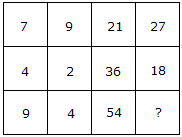# Verbal Reasoning - Character Puzzles - Discussion

### Discussion :: Character Puzzles - Character Puzzles 1 (Q.No.22)

22.

Which one will replace the question mark ?[A]. 18 [B]. 24 [C]. 36 [D]. 58

Explanation:

(7 x 3) = 21 and (9 x 3) = 27

and (4 x 9) = 36 and (2 x 9) = 18

Therefore (9 x 6) = 54 and (4 x 6) = 24.

 Rashmita said: (Nov 29, 2013) ANS - Option B. Explanation : (21/7)*9 = 27. (36/4)*2 = 18. (54/9)*4 = 24.

 Raghav Jee Sharma said: (Oct 17, 2015) I likes this site. So solve easily trick.

 Shivamrai said: (Sep 2, 2020) 21/7) * 9 = 27. (36/4) * 2 = 18. (54/9) * 4 = 24.